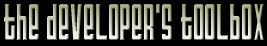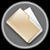This section of the archives stores flipcode's complete Developer Toolbox collection, featuring a variety of mini-articles and source code contributions from our readers.Q3A Maps And Curved Surfaces   Submitted byI implemented a small .bsp viewer in my engine and wondered about the squished look of pipes / cylindrical objekts in the level.

Normaly Q3A uses C1 bezier curves (3 controlpoints per line / 9 per patch)

The bezier function calculate a weight for each of the 3 control points from a value alpha [0..1]:

 ```weight1= (1-alpha)ÂÂ˛ weight2=2*(1-alpha)(alpha) weight3= alphaÂÂ˛ ```

But you can use a trigonometrical interpolation here, too.

The weights would now be the following:

 ```weight1= 1-cos(alpha*PI / 2); weight3= 1- sin(alpha*PI / 2); weight2= 1-weight1-weight3; ```

Now you will see really curved surfaces :).. and real cylinders

w.b.r.
M.Knuth

The zip file viewer built into the Developer Toolbox made use of the zlib library, as well as the zlibdll source additions.